Eric Smith

Introduction to Functions

Slide Duration:

Section 1: Properties of Real Numbers
Basic Types of Numbers

30m 41s

Intro
0:00
Objectives
0:07
Basic Types of Numbers
0:36
Natural Numbers
1:02
Whole Numbers
1:29
Integers
2:04
Rational Numbers
2:38
Irrational Numbers
5:06
Imaginary Numbers
6:48
Basic Types of Numbers Cont.
8:09
The Big Picture
8:10
Real vs. Imaginary Numbers
8:30
Rational vs. Irrational Numbers
8:48
Basic Types of Numbers Cont.
10:55
Number Line
11:06
Absolute Value
11:44
Inequalities
12:39
Example 1
13:16
Example 2
17:30
Example 3
21:56
Example 4
24:27
Example 5
27:48
Operations on Numbers

19m 26s

Intro
0:00
Objectives
0:06
Operations on Numbers
0:25
0:53
Subtraction
1:33
Multiplication & Division
2:19
Exponents
3:24
Bases
4:04
Square Roots
4:59
Principle Square Roots
5:09
Perfect Squares
6:32
Simplifying and Combining Roots
6:52
Example 1
8:16
Example 2
12:30
Example 3
14:02
Example 4
16:27
Order of Operations

12m 6s

Intro
0:00
Objectives
0:06
The Order of Operations
0:25
Work Inside Parentheses
0:42
Simplify Exponents
0:52
Multiplication & Division from Left to Right
0:57
Addition & Subtraction from Left to Right
1:11
Remember PEMDAS
1:21
The Order of Operations Cont.
2:27
Example
2:43
Example 1
3:55
Example 2
5:36
Example 3
7:35
Example 4
8:56
Properties of Real Numbers

18m 52s

Intro
0:00
Objectives
0:07
The Properties of Real Numbers
0:23
Commutative Property of Addition and Multiplication
0:44
Associative Property of Addition and Multiplication
1:50
Distributive Property of Multiplication Over Addition
3:20
Division Property of Zero
4:46
Division Property of One
5:23
Multiplication Property of Zero
5:56
Multiplication Property of One
6:17
6:29
Why Are These Properties Important?
6:53
Example 1
9:16
Example 2
13:04
Example 3
14:30
Example 4
16:57
Section 2: Linear Equations
The Vocabulary of Linear Equations

12m 22s

Intro
0:00
Objectives
0:09
The Vocabulary of Linear Equations
0:44
Variables
0:52
Terms
1:09
Coefficients
1:40
Like Terms
2:18
Examples of Like Terms
2:37
Expressions
4:01
Equations
4:26
Linear Equations
5:04
Solutions
5:55
Example 1
6:16
Example 2
7:16
Example 3
8:45
Example 4
10:20
Solving Linear Equations in One Variable

28m 52s

Intro
0:00
Objectives
0:08
Solving Linear Equations in One Variable
0:34
Conditional Cases
0:51
Identity Cases
1:09
1:30
Solving Linear Equations in One Variable Cont.
2:00
2:10
Multiplication Property of Equality
2:43
Steps to Solve Linear Equations
3:14
Example 1
4:22
Example 2
8:21
Example 3
12:32
Example 4
14:19
Example 5
17:25
Example 6
22:17
Solving Formulas

12m 2s

Intro
0:00
Objectives
0:06
Solving Formulas
0:18
Formulas
0:26
Use the Same Properties as Solving Linear Equations
1:36
1:55
Multiplication Property of Equality
1:58
Steps to Solve Formulas
2:43
Example 1
3:56
Example 2
6:09
Example 3
8:39
Applications of Linear Equations

28m 41s

Intro
0:00
Objectives
0:10
Applications of Linear Equations
0:43
The Six-Step Method to Solving Word Problems
0:55
Common Terms
3:12
Example 1
5:03
Example 2
9:40
Example 3
13:48
Example 4
17:58
Example 5
23:28
Applications of Linear Equations, Motion & Mixtures

24m 26s

Intro
0:00
Objectives
0:21
Motion and Mixtures
0:46
Motion Problems: Distance, Rate, and Time
1:06
Mixture Problems: Amount, Percent, and Total
1:27
The Table Method
1:58
The Beaker Method
3:38
Example 1
5:05
Example 2
9:44
Example 3
14:20
Example 4
19:13
Section 3: Graphing
Rectangular Coordinate System

22m 55s

Intro
0:00
Objectives
0:11
The Rectangular Coordinate System
0:39
The Cartesian Coordinate System
0:40
X-Axis
0:54
Y-Axis
1:04
Origin
1:11
1:26
Ordered Pairs
2:10
Example 1
2:55
The Rectangular Coordinate System Cont.
6:09
X-Intercept
6:45
Y-Intercept
6:55
Relation of X-Values and Y-Values
7:30
Example 2
11:03
Example 3
12:13
Example 4
14:10
Example 5
18:38
Slope & Graphing

27m 58s

Intro
0:00
Objectives
0:11
Slope and Graphing
0:48
Standard Form
1:14
Example 1
2:24
Slope and Graphing Cont.
4:58
Slope, m
5:07
Slope is Rise over Run
6:11
Don't Mix Up the Coordinates
8:20
Example 2
9:39
Slope and Graphing Cont.
14:26
Slope-Intercept Form
14:34
Example 3
16:55
Example 4
18:00
Slope and Graphing Cont.
19:00
Rewriting an Equation in Slope-Intercept Form
19:39
Rewriting an Equation in Standard Form
20:09
Slopes of Vertical & Horizontal Lines
20:56
Example 5
22:49
Example 6
24:09
Example 7
25:59
Example 8
26:57
Linear Equations in Two Variables

20m 36s

Intro
0:00
Objectives
0:13
Linear Equations in Two Variables
0:36
Point-Slope Form
1:07
Substitute in the Point and the Slope
2:21
Parallel Lines: Two Lines with the Same Slope
4:05
Perpendicular Lines: Slopes are Negative Reciprocals of Each Other
4:39
Perpendicular Lines: Product of Slopes is -1
5:24
Example 1
6:02
Example 2
7:50
Example 3
10:49
Example 4
13:26
Example 5
15:30
Example 6
17:43
Section 4: Functions
Introduction to Functions

21m 24s

Intro
0:00
Objectives
0:07
Introduction to Functions
0:58
Relations
1:03
Functions
1:37
Independent Variables
2:00
Dependent Variables
2:11
Function Notation
2:21
Function
3:43
Input and Output
3:53
Introduction to Functions Cont.
4:45
Domain
4:46
Range
4:55
Functions Represented by a Diagram
6:41
Natural Domain
9:11
Evaluating Functions
12:02
Example 1
13:13
Example 2
15:03
Example 3
16:18
Example 4
19:54
Graphing Functions

16m 12s

Intro
0:00
Objectives
0:09
Graphing Functions
0:54
Using Slope-Intercept Form
1:56
Vertical Line Test
2:58
Determining the Domain
4:20
Determining the Range
5:43
Example 1
6:06
Example 2
7:18
Example 3
8:31
Example 4
11:04
Section 5: Systems of Linear Equations
Systems of Linear Equations

25m 54s

Intro
0:00
Objectives
0:13
Systems of Linear Equations
0:46
System of Equations
0:51
System of Linear Equations
1:15
Solutions
1:35
Points as Solutions
1:53
Finding Solutions Graphically
5:13
Example 1
6:37
Example 2
12:07
Systems of Linear Equations Cont.
17:01
One Solution, No Solution, or Infinite Solutions
17:10
Example 3
18:31
Example 4
22:37
Solving a System Using Substitution

20m 1s

Intro
0:00
Objectives
0:09
Solving a System Using Substitution
0:32
Substitution Method
1:24
Substitution Example
2:35
One Solution, No Solution, or Infinite Solutions
7:50
Example 1
9:45
Example 2
12:48
Example 3
15:01
Example 4
17:30
Solving a System Using Elimination

19m 40s

Intro
0:00
Objectives
0:09
Solving a System Using Elimination
0:27
Elimination Method
0:42
Elimination Example
2:01
One Solution, No Solution, or Infinite Solutions
7:05
Example 1
8:53
Example 2
11:46
Example 3
15:37
Example 4
17:45
Applications of Systems of Equations

24m 34s

Intro
0:00
Objectives
0:12
Applications of Systems of Equations
0:30
Word Problems
1:31
Example 1
2:17
Example 2
7:55
Example 3
13:07
Example 4
17:15
Section 6: Inequalities
Solving Linear Inequalities in One Variable

17m 13s

Intro
0:00
Objectives
0:08
Solving Linear Inequalities in One Variable
0:37
Inequality Expressions
0:46
Linear Inequality Solution Notations
3:40
Inequalities
3:51
Interval Notation
4:04
Number Lines
4:43
Set Builder Notation
5:24
Use Same Techniques as Solving Equations
6:59
'Flip' the Sign when Multiplying or Dividing by a Negative Number
7:12
'Flip' Example
7:50
Example 1
8:54
Example 2
11:40
Example 3
14:01
Compound Inequalities

16m 13s

Intro
0:00
Objectives
0:07
Compound Inequalities
0:37
'And' vs. 'Or'
0:44
'And'
3:24
'Or'
3:35
'And' Symbol, or Intersection
3:51
'Or' Symbol, or Union
4:13
Inequalities
4:41
Example 1
6:22
Example 2
9:30
Example 3
11:27
Example 4
13:49
Solving Equations with Absolute Values

14m 12s

Intro
0:00
Objectives
0:08
Solve Equations with Absolute Values
0:18
Solve Equations with Absolute Values Cont.
1:11
Steps to Solving Equations with Absolute Values
2:21
Example 1
3:23
Example 2
6:34
Example 3
10:12
Inequalities with Absolute Values

17m 7s

Intro
0:00
Objectives
0:07
Inequalities with Absolute Values
0:23
Recall…
2:08
Example 1
3:39
Example 2
6:06
Example 3
8:14
Example 4
10:29
Example 5
13:29
Graphing Inequalities in Two Variables

15m 33s

Intro
0:00
Objectives
0:07
Graphing Inequalities in Two Variables
0:32
Split Graph into Two Regions
1:53
Graphing Inequalities
5:44
Test Points
6:20
Example 1
7:11
Example 2
10:17
Example 3
13:06
Systems of Inequalities

21m 13s

Intro
0:00
Objectives
0:08
Systems of Inequalities
0:24
Test Points
1:10
Steps to Solve Systems of Inequalities
1:25
Example 1
2:23
Example 2
7:28
Example 3
12:51
Section 7: Polynomials
Integer Exponents

44m 51s

Intro
0:00
Objectives
0:09
Integer Exponents
0:42
Exponents 'Package' Multiplication
1:25
Example 1
2:00
Example 2
3:13
Integer Exponents Cont.
4:50
Product Rule for Exponents
4:51
Example 3
7:16
Example 4
10:15
Integer Exponents Cont.
13:13
Power Rule for Exponents
13:14
Power Rule with Multiplication and Division
15:33
Example 5
16:18
Integer Exponents Cont.
20:04
Example 6
20:41
Integer Exponents Cont.
25:52
Zero Exponent Rule
25:53
Quotient Rule
28:24
Negative Exponents
30:14
Negative Exponent Rule
32:27
Example 7
34:05
Example 8
36:15
Example 9
39:33
Example 10
43:16

18m 33s

Intro
0:00
Objectives
0:07
0:25
Terms
0:33
Coefficients
0:51
1:13
Like Terms
1:29
Polynomials
2:21
Monomials, Binomials, Trinomials, and Polynomials
5:41
Degrees
7:00
Evaluating Polynomials
8:12
9:25
Example 1
11:48
Example 2
13:00
Example 3
14:41
Example 4
16:15
Multiplying Polynomials

25m 7s

Intro
0:00
Objectives
0:06
Multiplying Polynomials
0:41
Distributive Property
1:00
Example 1
2:49
Multiplying Polynomials Cont.
8:22
Organize Terms with a Table
8:23
Example 2
13:40
Multiplying Polynomials Cont.
16:33
Multiplying Binomials with FOIL
16:48
Example 3
18:49
Example 4
20:04
Example 5
21:42
Dividing Polynomials

44m 56s

Intro
0:00
Objectives
0:07
Dividing Polynomials
0:29
Dividing Polynomials by Monomials
2:10
Dividing Polynomials by Polynomials
2:59
Dividing Numbers
4:09
Dividing Polynomials Example
8:39
Example 1
12:35
Example 2
14:40
Example 3
16:45
Example 4
21:13
Example 5
24:33
Example 6
29:02
Dividing Polynomials with Synthetic Division Method
33:36
Example 7
38:43
Example 8
42:24
Section 8: Factoring Polynomials
Greatest Common Factor & Factor by Grouping

28m 27s

Intro
0:00
Objectives
0:09
Greatest Common Factor
0:31
Factoring
0:40
Greatest Common Factor (GCF)
1:48
GCF for Polynomials
3:28
Factoring Polynomials
6:45
Prime
8:21
Example 1
9:14
Factor by Grouping
14:30
Steps to Factor by Grouping
17:03
Example 2
17:43
Example 3
19:20
Example 4
20:41
Example 5
22:29
Example 6
26:11
Factoring Trinomials

21m 44s

Intro
0:00
Objectives
0:06
Factoring Trinomials
0:25
Recall FOIL
0:26
Factor a Trinomial by Reversing FOIL
1:52
Tips when Using Reverse FOIL
5:31
Example 1
7:04
Example 2
9:09
Example 3
11:15
Example 4
13:41
Factoring Trinomials Cont.
15:50
Example 5
18:42
Factoring Trinomials Using the AC Method

30m 9s

Intro
0:00
Objectives
0:08
Factoring Trinomials Using the AC Method
0:27
Factoring when Leading Term has Coefficient Other Than 1
1:07
Reversing FOIL
1:18
Example 1
1:46
Example 2
4:28
Factoring Trinomials Using the AC Method Cont.
7:45
The AC Method
8:03
Steps to Using the AC Method
8:19
Tips on Using the AC Method
9:29
Example 3
10:45
Example 4
16:50
Example 5
21:08
Example 6
24:58
Special Factoring Techniques

30m 14s

Intro
0:00
Objectives
0:07
Special Factoring Techniques
0:26
Difference of Squares
1:46
Perfect Square Trinomials
2:38
No Sum of Squares
3:32
Special Factoring Techniques Cont.
4:03
Difference of Squares Example
4:04
Perfect Square Trinomials Example
5:29
Example 1
7:31
Example 2
9:59
Example 3
11:47
Example 4
15:09
Special Factoring Techniques Cont.
19:07
Sum of Cubes and Difference of Cubes
19:08
Example 5
23:13
Example 6
26:12

23m 38s

Intro
0:00
Objectives
0:08
0:19
0:20
Zero Factor Property
1:39
Zero Factor Property Example
2:34
Example 1
4:00
Solving Quadratic Equations by Factoring Cont.
5:54
Example 2
7:28
Example 3
11:09
Example 4
14:22
Solving Quadratic Equations by Factoring Cont.
18:17
Higher Degree Polynomial Equations
18:18
Example 5
20:22

29m 27s

Intro
0:00
Objectives
0:12
0:29
Linear Factors
0:38
1:22
Principle of Square Roots
3:36
Completing the Square
4:50
Steps for Using Completing the Square
5:15
Completing the Square Works on All Quadratic Equations
6:41
7:28
Discriminants
8:25
10:11
Example 1
11:54
Example 2
13:03
Example 3
16:30
Example 4
21:29
Example 5
25:07

16m 47s

Intro
0:00
Objectives
0:08
0:24
Using a Substitution
0:53
U-Substitution
1:26
Example 1
2:07
Example 2
5:36
Example 3
8:31
Example 4
11:14

29m 4s

Intro
0:00
Objectives
0:09
0:35
Squared Variable
0:40
Principle of Square Roots
0:51
Example 1
1:09
Example 2
2:04
3:34
Example 3
4:42
Example 4
13:33
Example 5
20:50

26m 53s

Intro
0:00
Objectives
0:06
0:39
Axis of Symmetry
1:46
Vertex
2:12
Transformations
2:57
3:23
Example 1
5:06
Example 2
6:02
Example 3
9:07
11:26
Completing the Square
12:02
Vertex Shortcut
12:16
Example 4
13:49
Example 5
17:25
Example 6
20:07
Example 7
23:43
Polynomial Inequalities

21m 42s

Intro
0:00
Objectives
0:07
Polynomial Inequalities
0:30
Solving Polynomial Inequalities
1:20
Example 1
2:45
Polynomial Inequalities Cont.
5:12
Larger Polynomials
5:13
Positive or Negative Intervals
7:16
Example 2
9:01
Example 3
13:53
Section 10: Rational Equations
Multiply & Divide Rational Expressions

26m 41s

Intro
0:00
Objectives
0:09
Multiply and Divide Rational Expressions
0:44
Rational Numbers
0:55
Dividing by Zero
1:45
Canceling Extra Factors
2:43
Negative Signs in Fractions
4:52
Multiplying Fractions
6:26
Dividing Fractions
7:17
Example 1
8:04
Example 2
14:01
Example 3
16:23
Example 4
18:56
Example 5
22:43

20m 24s

Intro
0:00
Objectives
0:07
0:41
Common Denominators
0:52
Common Denominator Examples
1:14
Steps to Adding and Subtracting Rational Expressions
2:39
Example 1
3:34
Example 2
5:27
Adding and Subtracting Rational Expressions Cont.
6:57
Least Common Denominators
6:58
Transitioning from Fractions to Rational Expressions
9:08
Identifying Least Common Denominators for Rational Expressions
9:56
10:41
Example 3
11:19
Example 4
12:36
Example 5
15:08
Example 6
16:46
Complex Fractions

18m 23s

Intro
0:00
Objectives
0:09
Complex Fractions
0:37
Dividing to Simplify Complex Fractions
1:10
Example 1
2:03
Example 2
3:58
Complex Fractions Cont.
9:15
Using the Least Common Denominator to Simplify Complex Fractions
9:16
10:07
Example 3
10:42
Example 4
14:28
Solving Rational Equations

16m 24s

Intro
0:00
Objectives
0:07
Solving Rational Equations
0:23
Isolate the Specified Variable
1:23
Example 1
1:58
Example 2
5:00
Example 3
8:23
Example 4
13:25
Rational Inequalities

18m 54s

Intro
0:00
Objectives
0:06
Rational Inequalities
0:18
Testing Intervals for Rational Inequalities
0:38
Steps to Solving Rational Inequalities
1:05
Tips to Solving Rational Inequalities
2:27
Example 1
3:33
Example 2
12:21
Applications of Rational Expressions

20m 20s

Intro
0:00
Objectives
0:07
Applications of Rational Expressions
0:27
Work Problems
1:05
Example 1
2:58
Example 2
6:45
Example 3
13:17
Example 4
16:37
Variation & Proportion

27m 4s

Intro
0:00
Objectives
0:10
Variation and Proportion
0:34
Variation
0:35
Inverse Variation
1:01
Direct Variation
1:10
Setting Up Proportions
1:31
Example 1
2:27
Example 2
5:36
Variation and Proportion Cont.
8:29
Inverse Variation
8:30
Example 3
9:20
Variation and Proportion Cont.
12:41
Constant of Proportionality
12:42
Example 4
13:59
Variation and Proportion Cont.
16:17
Varies Directly as the nth Power
16:30
Varies Inversely as the nth Power
16:53
Varies Jointly
17:09
Combining Variation Models
17:36
Example 5
19:09
Example 6
22:10
Rational Exponents

14m 32s

Intro
0:00
Objectives
0:07
Rational Exponents
0:32
Power on Top, Root on Bottom
1:05
Example 1
1:37
Rational Exponents Cont.
4:04
Using Rules from Exponents for Radicals as Exponents
4:05
Combining Terms Under a Single Root
4:50
Example 2
5:21
Example 3
7:39
Example 4
11:23
Example 5
13:14
Simplify Rational Exponents

15m 12s

Intro
0:00
Objectives
0:07
Simplify Rational Exponents
0:25
0:26
Product Rule to Simplify Square Roots
1:11
1:42
Applications of Product and Quotient Rules
2:17
Higher Roots
2:48
Example 1
3:39
Example 2
6:35
Example 3
8:41
Example 4
11:09

17m 22s

Intro
0:00
Objectives
0:07
0:33
Like Terms
1:29
Bases and Exponents May be Different
2:02
Bases and Powers Must be Same when Adding and Subtracting
2:42
3:55
Example 1
4:47
Example 2
6:00
7:10
Simplify the Bases to Look the Same
7:25
Example 3
8:23
Example 4
11:45
Example 5
15:10

19m 24s

Intro
0:00
Objectives
0:08
0:25
0:26
1:11
Don’t Distribute Powers
2:54
4:25
Rationalizing Denominators
6:40
Example 1
7:22
Example 2
8:32
9:23
Rationalizing Denominators with Higher Roots
9:25
Example 3
10:51
Example 4
11:53
13:13
Rationalizing Denominators with Conjugates
13:14
Example 5
15:52
Example 6
17:25

15m 5s

Intro
0:00
Objectives
0:07
0:17
0:18
Isolate the Roots and Raise to Power
0:34
Example 1
1:13
Example 2
3:09
7:04
7:05
Example 3
7:54
Example 4
13:07
Complex Numbers

29m 16s

Intro
0:00
Objectives
0:06
Complex Numbers
1:05
Imaginary Numbers
1:08
Complex Numbers
2:27
Real Parts
2:48
Imaginary Parts
2:51
Commutative, Associative, and Distributive Properties
3:35
4:04
Multiplying Complex Numbers
6:16
Dividing Complex Numbers
8:59
Complex Conjugate
9:07
Simplifying Powers of i
14:34
Shortcut for Simplifying Powers of i
18:33
Example 1
21:14
Example 2
22:15
Example 3
23:38
Example 4
26:33
Bookmark & Share Embed

## Copy & Paste this embed code into your website’s HTML

Please ensure that your website editor is in text mode when you paste the code.
(In Wordpress, the mode button is on the top right corner.)
×
• - Allow users to view the embedded video in full-size.
Since this lesson is not free, only the preview will appear on your website.

• ## Related Books0 answersPost by Leo Jiang on July 14, 2018In the practice question "R = {(1, 1), (4, 2), (5, 6), (3, 0)}. Represent R as a table and as a graph.", the solution graphs (3, 0) as (0, 0). Can someone please fix this?1 answerLast reply by: Professor Eric SmithWed Jul 16, 2014 8:02 PMPost by Anura Hewagama on July 14, 2014What would happen if you used brackets instead of parentheses for infinity?1 answerLast reply by: Professor Eric SmithSun Jul 6, 2014 2:30 PMPost by patrick guerin on July 3, 2014Is there an easy way to keep domain and range straight?

### Introduction to Functions

• A function is a special type of relation, such that for every x value there is one and only one y value.
• When writing functions we usually write out the name of the function, followed by the independent variable in parenthesis, then the expression or rule that defines the function.
• The domain of a function contains all possible inputs, and the range of a function contains all possible outputs.
• If no domain is specified, then the natural domain is used. This contains all real numbers that do not cause the function to be undefined.
• To evaluate a function, we can substitute a number in for the independent variable.

### Introduction to Functions

Let the relation R = { (1,1),(4,2),(5,6),(3,0)} . Represent R as a table and as a graph
• Find the x and y values
•  x
 line
 1
 4
 5
 3
 y
 line
 1
 2
 6
 0
• Chart the values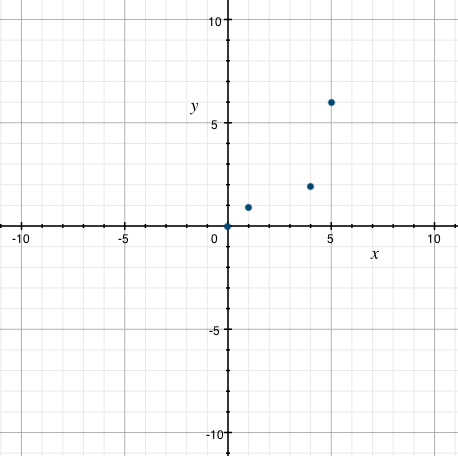Let the relation R = { ( − 1,9.5),(5,0),( − 3, − 6),(8.5, − 7)} . Represent R as a table and as a graph
• Find the x and y values
•  x
 line
 − 1
 5
 − 3
 8.5
 y
 line
 9.5
 0
 − 6
 − 7
• Chart the values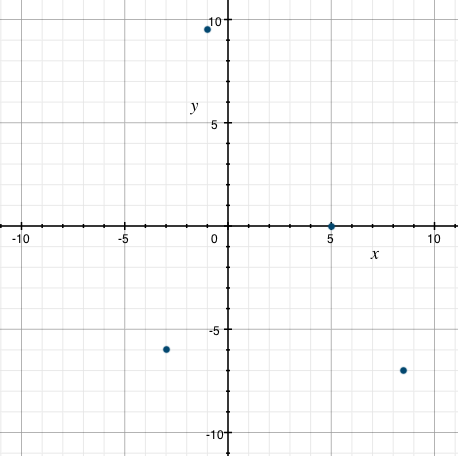Determine the values of the relation R from the figure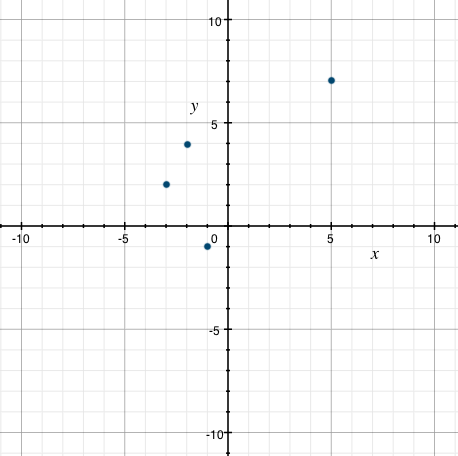• Find the x and y values
•  x
 line
 − 1
 − 2
 − 3
 5
 y
 line
 − 1
 4
 2
 7
• Create the set from the coordinate pairs
R = { ( − 1, − 1),( − 2,4),( − 3,2),(5,7)}
Determine the values of the relation R from the figure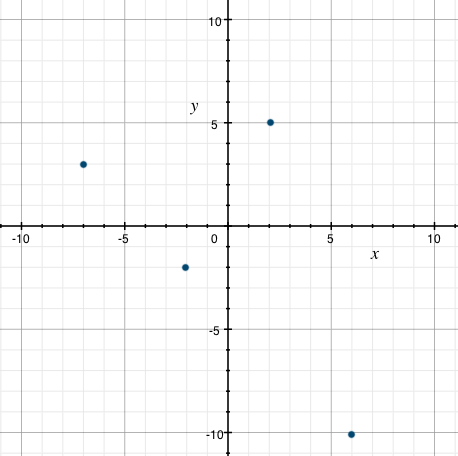• Find the x and y values
 x
 line
 − 7
 − 2
 2
 6
 y
 line
 3
 − 2
 5
 − 10

• Create the set from the coordinate pairs
R = { ( − 7,3),( − 2, − 2),(2,5),( − 6,10)}
The relation R is described by the following table. Find the domain and range of R.
 x
 line
 − 10
 1
 3
 5
 y
 line
 3
 − 2
 5
 − 10
Domain correspond to x values and y values correspond to the range
Domain = { − 10,1,3,5}
Range = { 3, − 2,5, − 10}
The relation R is described by the following table. Find the domain and range of R.
 x
 line
 2
 4
 5
 − 9
 y
 line
 1
 − 2
 − 4
 3
Domain correspond to x values and y values correspond to the range
Domain = { 2,4,5, − 9}
Range = { 1, − 2, − 4, − 3}
Determine the domain and range of the relation R represented by the graph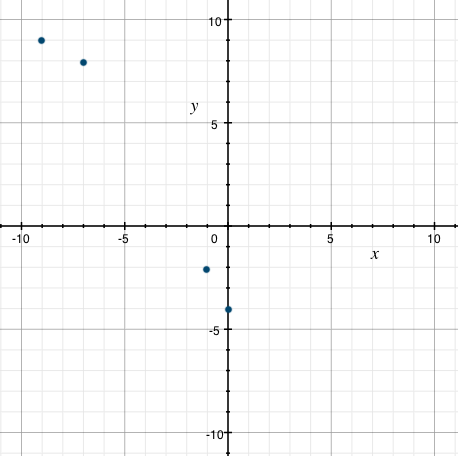Domain correspond to x values and y values correspond to the range
Domain = { − 9, − 7, − 1,0}
Range = { 9,8, − 2, − 4}
Determine the domain and range of the relation R represented by the graph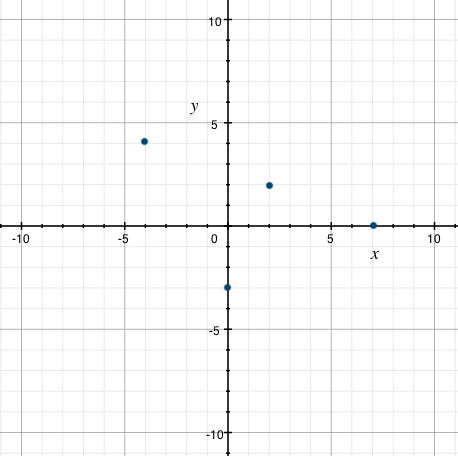Domain correspond to x values and y values correspond to the range
Domain = { − 4,0,2,7}
Range = { 4, − 3,2,0}
The relation R is given by the following table. Write the inverse of R as a list of ordered pairs,then find the domain and range of the inverse of R.
 x
 line
 − 2
 − 1
 0
 5
 y
 line
 9
 8
 7
 6
• The inverse has the y and x values switched
• Inverse of R = { (9, - 2),(8, - 1),(7,0),(6,5)}
Domain correspond to x values and y values correspond to the range of the inverse
Domain = { 9,8,7,6}
Range = { − 2, − 1,0,5}
The relation R is given by the following table. Write the inverse of R as a list of ordered pairs, then find the domain and range of the inverse of R.
 x
 line
 − 10
 3
 − 4
 7
 y
 line
 4
 0
 − 2
 − 6
• The inverse has the y and x values switched
• Inverse of R = { (4, - 10),(0,3),( - 2, - 4),( - 6,7)}
Domain correspond to x values and y values correspond to the range of the inverse
Domain = { 9,8,7,6}
Range = { − 10,3, − 4,7}
Is the relation a function?
{ (3,0), ( − 4,2), ( − 2,1), ( − 1,3), (0,4),( − 2, − 1)}
• Domain: { 3, − 4, − 2, − 1,0, − 2}
• Domain: { 3, − 4,, − 1,0,}
Not a function
Is the relation a function?
{ (1,4), (2, − 1), (0,3), ( − 3,1), ( − 1,2),( − 3, − 2)}
• Domain:{ 1,2,0, − 3, − 1, − 3}
• Domain: { 1,2,0,, − 1,}
Not a function
Is the relation a function?
{ ( − 1, − 1), (0, − 3), (3,0), ( − 3,1), (1,2),(2, − 2)}
• Domain: { − 1,0,3, − 3,1,2}
Yes, this is a function.
Let f(x) = − 2x + 8
Find f(3)
• f(3) = − 2(3) + 8
• f(3) = − 6 + 8
f(3) = 2
Let f(x) = − 7x + 8
Find f(6) − 9
• f(6) = [ − 7(6) + 8] − 9
• f(6) = [ − 42 + 8] − 9
• f(6) = − 33 − 9
f(6) = − 42
Let g(x) = − 5x2 + 11x − 15
Find 2g( − 2)
• g( − 2) = − 5( − 2)2 + 11( − 2) − 15
• g( − 2) = − 5(4) + 11( − 2) − 15
• g( − 2) = − 20 − 22 − 15
• g( − 2) = − 55
• 2g( − 2) = 2( − 55)
- 110
Let g(x) = − 3x3 − 4x + 5
Find 6g(2) + 2g(1)
• g(2) = − 3(2)3 − 4(2) + 5
• g(2) = − 3(8) − 4(2) + 5
• g(2) = − 24 − 8 + 5
• g(2) = − 27
• g(1) = − 3(1)3 − 4(1) + 5
• g(1) = − 3(1) − 4(1) + 5
• g(1) = − 3 − 4 + 5
• g(1) = − 2
• 6g(2) + 2g(1)
• 6( − 27) + 2( − 2)
− 162 − 4 = − 166
Let g(x) = 2x2 + 5
Find g(2) + 10g(1)
• g(2) = 2(2)2 + 5
= 2*4 + 5
= 8 + 5
= 13
• 10g(1) = 10[2(1)2 + 5]
= 10[2*1 + 5]
= 10(2 + 5)
= 70
g(2) + 10g(1) = 13 + 70 = 83
Do these points represent a function?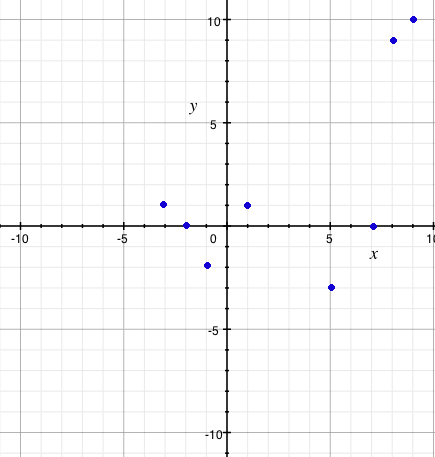• Use the Vertical Line Test
Yes, because there are no two or more points in the same vertical line.
Do these points represent a function?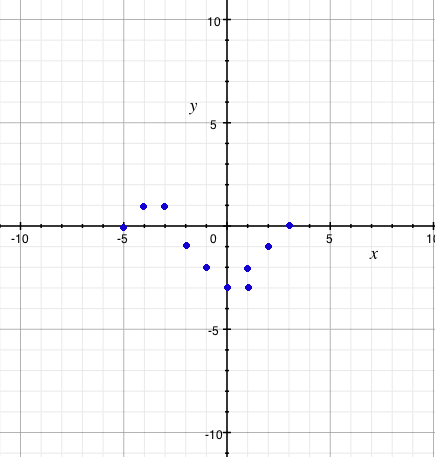• Use the Vertical Line Test
No because it fails two or more points are on the same vertical line, thus having multiple values for the range.

*These practice questions are only helpful when you work on them offline on a piece of paper and then use the solution steps function to check your answer.

### Introduction to Functions

Lecture Slides are screen-captured images of important points in the lecture. Students can download and print out these lecture slide images to do practice problems as well as take notes while watching the lecture.

• Intro 0:00
• Objectives 0:07
• Introduction to Functions 0:58
• Relations
• Functions
• Independent Variables
• Dependent Variables
• Function Notation
• Function 3:43
• Input and Output
• Introduction to Functions Cont. 4:45
• Domain
• Range
• Functions Represented by a Diagram
• Natural Domain
• Evaluating Functions
• Example 1 13:13
• Example 2 15:03
• Example 3 16:18
• Example 4 19:54

### Transcription: Introduction to Functions

Welcome back to www.educator.com.0000

In this lesson we are going to take a look at an introduction to functions.0002

There is quite a bit to cover when it comes to functions, we will go over this bit by bit.0008

Some of the first things I will do with functions is look at some of the terms.0014

In other words, what exactly makes a function and why is it a special type of a relationship.0019

What is it that makes a function a function?0024

We will get into a term such as the independent and dependent variable so they can find those parts in a function.0028

We will talk about how functions can be like little machines and how they have input and output.0035

We will call this out its domain and its range.0040

We will get into a little bit on how you can represent function using diagrams that we can better keep track of the inputs and outputs0044

and also how you can work with functions by evaluating them.0051

Let us take a look.0056

A function is a very special type of relation.0061

What a relation does is it connects 2 variables and usually we use a pair of ordered pairs to describe that connection.0065

Maybe I have an x variable and a y variable and one other connected.0074

I can show you that anytime I use a 2 for x, I get a 3 for y.0078

There are many other types of connections that you can consider as part of a relation.0084

Some of our equations can be considered a type of relation.0091

A function is just a special kind of one of these relations.0097

It is special because for every x value it has, there is one and only one y value associated with it.0101

What that means is that if you use a particular x value, you are never going to get two different values using that one x value.0111

When we represent functions, we usually identify some sort of independent variable.0120

This is what we get to choose for in terms of the x.0126

The dependent variable is usually represented by y and it depends on whatever we choose for x.0131

Some of the notation you may see for functions looks like this.0142

It is important that we can pick out each of the different parts here.0147

The first thing is what is this f out in front? f represents the name of the function.0152

This function is called f.0159

The x inside the parentheses right next to it is not multiplication, what that is indicating is what the variable is.0168

We have our function called f and it takes a variable of x.0180

Everything after that on the other side, that represents the relation or how things are connected.0186

You can also consider this as the rule of the function or what it does when you use a particular x.0196

The way you read this notation is f(x) = 3x + 9.0204

Our f part helps us identify what variables are being used inside the function.0215

To better understand what type of relations our function is, I like to tell my students that they are a lot like little machines.0226

They take some input and you put into and they produce some output.0233

A nice little diagram would look something like this down here.0240

Maybe my function f here takes an input of 3 and produces an output of 5.0246

If we start to gather up together more of its inputs and outputs, we can represent those as a whole bunch of ordered pairs.0254

What this list of ordered pairs would say is that my function takes an input of 1 and produces an output of 2.0262

If I give it an input of 3 then I get an output of 5.0272

Every ordered pair is telling me what the input and output was for that particular value.0278

Now, if we collected together all of the inputs, this would be known as the domain of the function.0288

If we collected together all of the outputs of the function, this be known as the range.0294

Using the example from a whole list of ordered pairs, we can actually identify all the things used as an input and all the things used as an output.0301

Remember this first value here, those are our x’s, those are inputs and the second values, those are our outputs.0311

Let us simply just list out all of the inputs and call that our domain and list out all the outputs and call that our range.0329

We used 1, 3, 4, 7 for input and what we got as output was 3, -2, 5, 10.0336

You can also represent a relation in the same way, so it is important to point out that this particular relation here is actually a function.0351

The reason why we know it is a function is that every time we are looking at any particular output, it only has one output associated with it.0360

Input of 1 output of 3, input of 3 output of -2.0370

You do not see anything on this list like 1, 7 because that would be a problem0377

because the 1 would go to 3 and 1 would go to 7, you will get 2 outputs.0384

I just want to make a little note here that this is a function because it satisfies our definition.0390

A great way to visualize what is happening with the inputs and outputs is to use a diagram.0403

One way to do that is to list all of your domains and group it together in a circle or a box.0409

And you group together all of your range in the same way.0415

You can show the relation by using arrows to connect the two.0419

That is exactly what I have done down below.0423

Here I have two different relations, you can see my inputs and you can see my outputs.0426

We will consider these our domain and our range.0436

The big question is does it satisfy the definition of a function or is it just simply some relation.0443

Let us take a look at it and see what happens to those inputs and outputs.0449

Remember, it is a function if every input you give it goes to exactly 1 output.0453

Let us see what we got.0459

Looking at this input here of 1, it looks like it has an output of 4 and that is the only thing it has for now, I will put just 4.0461

Let us check out the 3, if I use an input of 3 it looks like I will get an output of 4 and I will get an output of 0.0470

Let me highlight that.0479

Notice how this one has an arrow that goes right over here to 4 and has another one that goes out to 0.0483

When I use an input of 3, it goes to two different outputs then I can say that this is not a function.0493

It is close but it does not work.0509

Since we know that one was not a function, let us take a look at the other relation.0512

When I use an input of 1 it goes to 8, I will use an input of 3 it goes to 5, input of 6 goes to 1, input of 7 goes to 0.0519

Every time I use an input here, it only goes to one output.0530

This one was okay, this one is a function.0534

You can see these diagrams help out with looking at what is in the domain and range, it is all grouped together nice and simple.0542

In some functions, they may not actually specify what domain is being used and do not actually says what the domain is.0555

We just assume that is known as the natural domain.0563

What this natural domain represents is all of the possible real numbers that you could use as input0567

as long as it does not make the function itself undefined.0574

You might start with thinking about all possible numbers and eliminating ones that you can not use.0578

To help better identify what you can not use in the domain, look for stuff like fractions or even roots.0586

The reason for this is, you cannot divide by 0.0595

If any value would make the bottom of a fraction 0 then you toss them out, they are not in the domain.0600

Also, we do not want to have to deal with imaginary numbers, we do not want negative numbers underneath an even root.0607

If that happens, we will toss those out of the domain as well.0615

When it comes to writing down a lot of different numbers in the domain, there is a special way that we go about doing that.0620

We often use what is known as interval notation.0628

What interval notation is, it is a way of packaging up all of the range of numbers on a number line.0631

Let me give you a nice quick example.0640

I wanted to describe all the numbers between 2 and 3.0641

I could do that by shading in those numbers on a number line so you can see that I have shaded in 2, 3, and everything in between.0647

To use interval notation to do this, I would list out the starting point and the endpoint of everything that I have shaded.0658

Then I would use a bracket to say that the endpoints are included.0670

Everything between 2 and 3, I’m using that square bracket because 2 and 3 shall also be included.0676

If we can go all the way up to a point but maybe not included, we will still use interval notation for this.0685

But usually we use a parenthesis when it is not included.0698

I have re adjusted the number line here, now I shaded everything from to 2 to 3 but not including 3.0704

I have made the corresponding interval notation to reflect that.0711

I started at 2 stop at 3, includes 2, but does not include 3.0715

How we work with these functions?0724

One of the more common things we do with functions is to simply evaluate them.0726

To evaluate a function, what we simply do is substitute in the value given in place of x.0734

If I'm dealing with this function f(x) = 3x + 9 and maybe I want to evaluate it at the number 7.0742

The way I do this is I just replace all x’s with 7.0750

You will see that the process itself is not that difficult.0759

It is sometimes just the notation that throws people off because when you look at the left side over here,0763

your brain sometimes looks at that and you want to think of multiplication, but it is not multiplication.0769

When you have that f(7), what you are saying is that you used an input of 7 and then over here is what you got as your output.0776

Keep that in mind when you are evaluating functions.0789

Let us get into some examples and see if we can figure out whether certain relations are functions0795

and maybe identify a domain, range, and all that fun stuff.0803

In this relation, I can see that my inputs would be the first values and my outputs those are the second values.0808

Let us represent this using a diagram just to help out.0820

We will group together all of our inputs into a giant circle over here.0824

We will group together all of our outputs into a giant circle over here.0828

First the input, I have -3, 6, I have another -3 so I do not want to list it twice and I have 5.0834

Our outputs are 7, -2, 4, and 9.0845

When I use an input of -3 it goes over here to 7 and when I use an input of 6, this heads over to -2.0853

I have another -3, -3 also goes to 4 and 5 goes to 9.0866

That is a bit of a problem isn’t it?0877

Notice how we have a single input and it goes to two different spots.0879

If we have a single input going to 2 outputs then we can say that this is not a function.0887

Here I see another diagram.0905

Let us see if we can figure out if this one is a function, 13 goes to 7, 81 goes to 7, 32 goes to 60, 27 goes to 19 and 45 goes to 55.0906

This one actually looks pretty good.0920

This is a function.0926

There is an interesting feature here that I want to point out,0932

you noticed in the last one we had one input that went to 2 different outputs and that made it not a function.0937

You may be curious if this would also make it not a function? After all, 13 and 81 go to the same spot.0943

That is okay, you can have two inputs go to the same spot0954

but what you do not want to happen is to have one input split off into two different places.0958

This right here, this is okay.0965

Let us see if we can identify the domain of a function just from looking at its equation.0980

Since no domain is specified, we are going to look for the natural domain.0986

That means we will assume that all real numbers can be used unless it makes the function undefined.0990

In these examples, I put one with a fraction because we want be concerned about dividing by 0.0997

I put another one with a square root because we do not want negatives underneath an even root, like the square root.1002

In this first one, the way that it would not work out is if I had a 0 in the bottom.1010

Are there any restricted values for x?1019

Anything can x can not be would that give us a 0.1022

Well, if I had to solve just the bottom part, I can see that if x was equal to -1 I would definitely have a problem.1026

I think that is the only issue that we would end up with.1036

Let us just write them out, x can not be -1.1039

Anything else is perfectly acceptable.1047

If I was looking at a number line and trying to shade in all the different things x could or could not be,1052

the number line would look something like this.1059

It could definitely be any of these negative values over here.1064

I’m digging a big open hole at -1 since it can not be that and it could be anything greater than that.1069

It can be any value but not just -1.1076

I will represent that using some intervals.1080

It could be anything from negative infinity all the way up to -1, I would not include that.1085

It can be anything from -1 up to infinity, I will use little u’s to connect those.1089

It can be anything just not -1.1096

Let us try another one, f(x) = √x+ + 5.1100

I want to make sure that whatever x + 5 is, we do not want it to be negative.1108

It is okay if it is 0 but definitely we do not want it to be negative.1115

The only way it would end up being negative is if x was less than -5.1126

Let us say x can not be less than -5.1134

We will represent this on a number line as well so we can end up making an interval for it.1147

Let us see, here I have -5, -4, -3, -2, -1, 0, I have some things that are less than -5.1153

x can be anything, it can even be -5 because you can take the square root of 0, it can be anything greater.1169

The domain of this one, the natural domain would be from -5 up to infinity and it is okay to include that -5.1182

For this one, we want to work on evaluating the function for some given values of x.1195

Be careful on this notation over here, we are not multiplying or simply substituting 3 into our function and seeing what the result is.1201

I'm going to replace everywhere I see an x with this number 3.1215

Once I substituted it in there, we go through and clean it up a bit, I have -12 + 9 or -3.1224

Our input was 3 and our output is -3.1237

Let us do the same thing for f(-5).1243

Same function, we will just put in a different value, -5.1248

We will work to simplify it.1257

-4 × -5, negative × negative will be positive, 4 × 5 is 20, 20 + 9 and we would get 29.1260

In evaluating functions, just simply substitute a value in there for x and end up simplifying it.1274

That is all I have for now, thank you for watching www.educator.com.1281

OR

### Start Learning Now

Our free lessons will get you started (Adobe Flash® required).